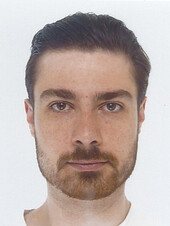# People

 Alexis Roquefeuil PositionTodai Postdoctoral Research Fellow (from 2019/10/02 ) [JSPS Fellow] Research FieldMathematics Mathematics E-MailLast Update 2020/08/04

Enumerative geometry is a branch of mathematics which essentially deals with counting the number of solutions to a geometrical problem, e.g. how many lines in the plane go through two points. In algebraic geometry, Gromov-Witten invariants are rational numbers which can sometimes be interpreted as the number of curves inside an ambient space meeting some incidence conditions. One way to define these invariants is to understand them as some intersection product on the moduli space parametrising such curves: M. Kontsevich’s moduli stack of stable maps. However, due to the highly non trivial geometry of this moduli space, a direct computation of these invariants seems quite difficult in general. A solution to produce computation is string theory’s mirror symmetry phenomenon. One way to interpret this phenomenon is as an isomorphism between two differential modules: the first encodes the Gromov-Witten invariants of our ambient space but is not so computable; the second is more explicit and comes from the singularity theory of another space, called the mirror of our ambient space.

More recently, A. Givental and Y.P. Lee defined in 2001 new invariants, called K-theoretic Gromov-Witten invariants, by replacing the cohomological constructions used in the definition of Gromov-Witten invariants by their K-theoretical analogues. I aim to understand how these two theories are related and how mirror symmetry can be applied to the new invariants.

Back to Member List.